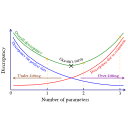Subsections

statistics.aicSynopsis

Calculate and store Akaike's Information Criterion (AIC) for each model.

statistics.aic()

Description

This will perform a calculation to obtain the chi-squared statistic for the current parameter values for each model, count the number of parameters per model and calculate Akaike's Information Criterion (AIC) using the formula AIC = chi2 + 2k. The AIC values, chi-squared values, and number of parameters will be stored in the appropriate location for the model in the relax data store.

The relax user manual (PDF), created 2020-08-26.